Force on a Current Carrying Conductor & Fleming's Left & Right Hand Rule

# Force on a Current Carrying Conductor & Fleming's Left & Right Hand Rule Notes | Study Science Class 10 - Class 10

## Document Description: Force on a Current Carrying Conductor & Fleming's Left & Right Hand Rule for Class 10 2022 is part of Science Class 10 preparation. The notes and questions for Force on a Current Carrying Conductor & Fleming's Left & Right Hand Rule have been prepared according to the Class 10 exam syllabus. Information about Force on a Current Carrying Conductor & Fleming's Left & Right Hand Rule covers topics like FORCE ON A CURRENT CARRYING CONDUCTOR PLACED IN A MAGNETIC FIELD&nbsp;, Fleming's Left Hand Rule&nbsp;, ELECTROMAGNETIC INDUCTION and Force on a Current Carrying Conductor & Fleming's Left & Right Hand Rule Example, for Class 10 2022 Exam. Find important definitions, questions, notes, meanings, examples, exercises and tests below for Force on a Current Carrying Conductor & Fleming's Left & Right Hand Rule.

Introduction of Force on a Current Carrying Conductor & Fleming's Left & Right Hand Rule in English is available as part of our Science Class 10 for Class 10 & Force on a Current Carrying Conductor & Fleming's Left & Right Hand Rule in Hindi for Science Class 10 course. Download more important topics related with notes, lectures and mock test series for Class 10 Exam by signing up for free. Class 10: Force on a Current Carrying Conductor & Fleming's Left & Right Hand Rule Notes | Study Science Class 10 - Class 10
 Table of contents FORCE ON A CURRENT CARRYING CONDUCTOR PLACED IN A MAGNETIC FIELD Fleming's Left Hand Rule ELECTROMAGNETIC INDUCTION
 1 Crore+ students have signed up on EduRev. Have you?

FORCE ON A CURRENT CARRYING CONDUCTOR PLACED IN A MAGNETIC FIELD
A current carrying conductor produces a magnetic field around it. When it is placed in a magnetic field, the two magnetic fields interact with each other and a net force acts on the conductor.

If the conductor of length l, carrying current I is lying inside a magnetic field of intensity B and is making an angle q with it, then force acting on the conductor is given by

F = IlB sin Θ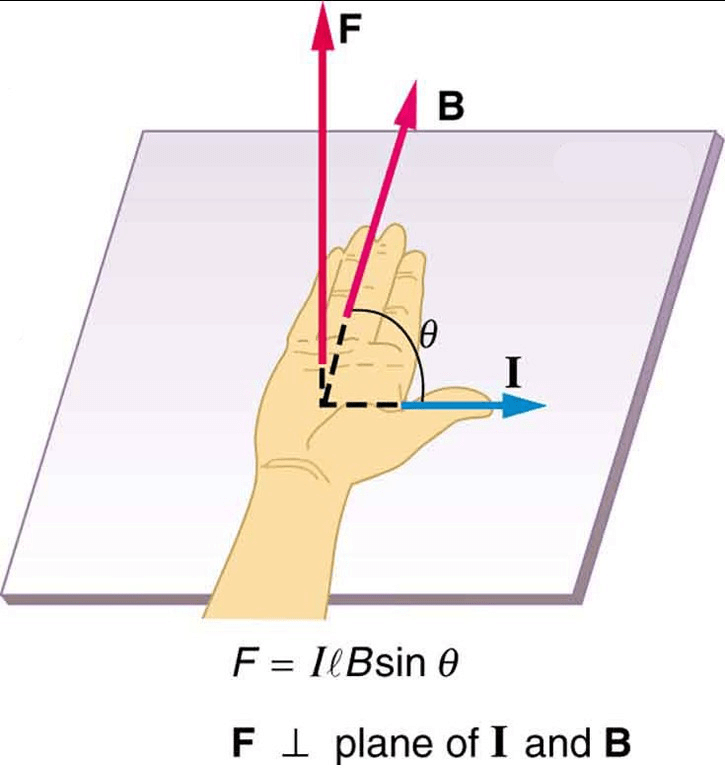If the conductor is lying perpendicular to the magnetic field, then

θ = 90° [sin θ = 1] and the force becomes F = IlB.

This force acts in a direction which is perpendicular to the plane containing the conductor and the magnetic field (Fig.) and is maximum.

If the conductor is lying parallel to the magnetic field, then θ = 0° (sin θ = 0) and the force becomes zero and is minimum.

Fleming's Left Hand Rule
Direction of the force acting on a current carrying conductor when placed in a magnetic field is given by Fleming's left hand rule, which states that:

If the forefinger, second finger and thumb of the left hand are stretched at right angles to each other, with the forefinger in the direction of the field and the second finger in the direction of the current then the thumb indicates the direction of the force.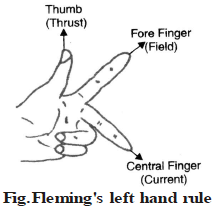Factors on which the force acting on the current carrying conductor depends

The force acting on a current carrying conductor is placed in the magnetic field depends upon :

(i) The strength of the magnetic field : If the conductor is placed in a strong magnetic field, it experiences a large force. That is, F∝ B (strength of magnetic field)

(ii) The strength of the electric curent : If large current flows through the conductor placed in the magnetic field, it experiences a large force. That is F ∝ I.

(iii) The length of the conductor : A long conductor experiences a greater force than the short conductor, when placed in the magnetic field. That is, F ∝ ℓ.

That is F∝ BIℓ

or F = kBIl

If k = 1, F = BIℓ

then B =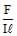If I = 1amp. and l = 1m then B = F

Thus, magnetic field strength (B) is defined as the force acting per unit current per unit length of a conductor placed perpendicular to the direction of the magnetic field.

SI unit of magnetic field strength is Tesla.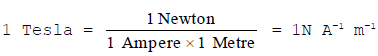ELECTROMAGNETIC INDUCTION

The phenomenon in which an electric current is induced in a circuit because of a changing magnetic field is called electromagnetic induction.
When a straight metallic wire is moved up and down in a magnetic field between the two poles of a horse shoe magnet then magnetic flux (number of magnetic lines of force) linked with the wire changes and an electric current is produced in the wire. A galvanometer connected across the two ends of the wire shows some deflection indicating that some current is produced. This current lasts only as long as the change in the flux continues.

This phenomenon is called electromagnetic induction. In short we can say that electromagnetic induction means production of electricity from magnetism. The electric current produced, is called induced current. The e.m.f. which produces this current, is called induced e.m.f.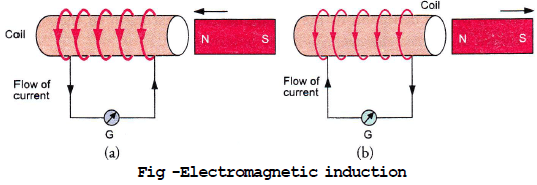Fig -Electromagnetic induction

Factors on which induced current depends

The value of induced current depends upon :

(i) The number of turns in the coil : If coil has large number of turns, then large induced current is produced in the closed coil.

(ii) The strength of the magnet : A strong magnet moved towards or away from the closed coil produced a large induced current.

(iii) The speed with which the magnet moves towards the coil : If the magnet moves very quickly, then large induced curent is produced in the closed coil.
FARADAY'S DISCOVERY OF ELECTROMAGNETIC INDUCTION
Michael Faraday in 1831, discovered phenomenon of electromagnetic induction. His experimental set up is shown in fig.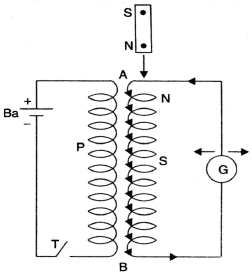When north pole of a magnet was held above end A of secondary coil S, no deflection was seen in galvanometer G. As the pole was moved towards the coil, galvanometer showed some deflection to the right. When magnet was left inside coil, galvanometer deflection became zero. When he took the magnet out of the coil, deflection in the galvanometer to the left side was observed. Hence current flow only when magnet was in motion and not when it was at rest near the coil or inside the coil.

Faraday performed a similar experiment by putting a primary coil P near coil AB. He completed primary circuit with a battery Ba and tapping key T.

So long as key was open, no deflection was seen in the galvanometer G. As the key was pressed and primary circuit was closed, galvanometer

showed some deflection to the right. Deflection became zero when key was kept pressed. When key was left to open, deflection in the galvanometer to the left side was observed.

The closing and opening of circuit changed the current in primary coil, which produced changing magnetic flux in the primary. This changing magnetic flux becomes linked with secondary and produces induced current (just as done by moving magnet), in the secondary.

Direction of the induced current is given by Fleming Right Hand rule which states that Stretch the thumb, the fore finger and the central finger of right hand in such a way that they are mutually perpendicular to each other. Then, if fore finger represents direction of Field and thumb represents direction of Motion of the conductor, then Central finger will represent the direction of induced Current (fig.).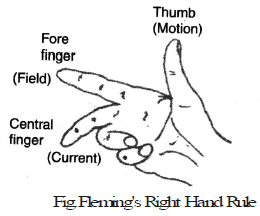The document Force on a Current Carrying Conductor & Fleming's Left & Right Hand Rule Notes | Study Science Class 10 - Class 10 is a part of the Class 10 Course Science Class 10.
All you need of Class 10 at this link: Class 10

## Science Class 10

83 videos|341 docs|162 tests
 Use Code STAYHOME200 and get INR 200 additional OFF

## Science Class 10

83 videos|341 docs|162 tests

Track your progress, build streaks, highlight & save important lessons and more!

,

,

,

,

,

,

,

,

,

,

,

,

,

,

,

,

,

,

,

,

,

;